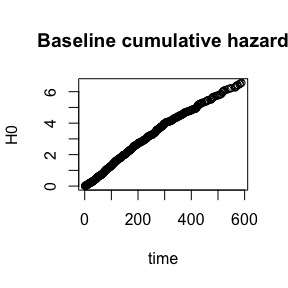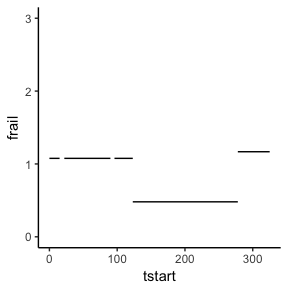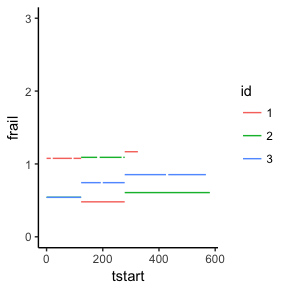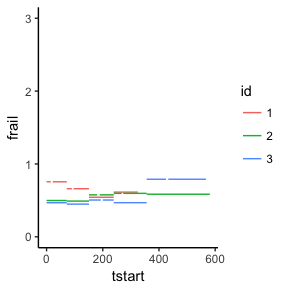# Fitting dynamic frailty models with dynfrail

## Package info

This is an R package for fitting semiparametric dynamic frailty models with the EM algorithm. The hazard for individual $$j$$ from cluster $$i$$ is specified as: $\lambda_{ij}(t | Z_i(t)) = Z_i(t) \exp(\beta^\top x_{ij}(t)) \lambda_0(t).$ The model used here is described in detail in Putter & van Houwelingen (2015). The distribution of $$Z_i(t)$$ is described by two parameters: $$\theta$$, that is an inverse-variability parameter of $$Z_i(t)$$ for a fixed $$t$$, and $$\lambda$$, that describes the autocorrelation of the process, so that for $$t_1 \leq t_2$$ $\mathrm{cor}(Z_i(t_1), Z_i(t_2)) = \exp(\lambda (t_2 - t_1)).$

The estimation process is that for fixed $$(\theta, \lambda)$$ the maximized profile likelihood is calculated, i.e. maximized with respect to $$(\beta, \lambda_0)$$. This profile likelihood is finally maximized itself.

## Installation

The development version from GitHub:

devtools::install_github("tbalan/dynfrail")

The following packages are needed to build dynfrail:

install.packages(c("RcppArmadillo", "tibble", "magrittr", "dplyr", "tidyr"))

The functioning of the package is described in the documentation of the main fitting function, dynfrail().

## Features

• gamma, PVF, compount Poisson, inverse Gaussian distributions
• flexible adjustment of estimation parameters
• semiparametric $$Z(t)$$ that changes values at every $$t$$ or piecewise constant $$Z(t)$$
• clustered survival data & recurrent events (calendar time or gaptime) ar supported

## Functions

• dynfrail() has a friendly syntax very similar to the frailtyEM package: next to a formula and data argument, the distribution argument is used to specify the distribution parameters and the control parameter is used for controling the precision of the estimation.
• dynfrail_prep() and dynfrail_fit() are used internally by dynfrail() but are made user-available. The first one prepares the input of dynfrail() to make it suitable for the actual EM algorithm. The second one performs one EM algorithm for fixed $$(\theta, \lambda)$$ to estimate the maximum ($$\beta$$, $$\lambda_0$$).

## Limitations

• slow even for medium sized data sets. It is recommended to start with a small number of piecewise constant intervals and/or a subset of the data
• no direct standard errors for $$(\theta, \lambda)$$.

## Analyzing the output

We take the asthma data set in the parfm package. I take a subset where each individual is censored after 3 events.

library(parfm)
library(dplyr)
data(asthma)

small_asthma <-
asthma %>%
group_by(Patid) %>%
mutate(linenr = 1:n()) %>%
filter(linenr <= 3)

This is a recurrent events data set in Andersen-Gill format with one covariate (Drug).

head(small_asthma)
## # A tibble: 6 x 7
## # Groups:   Patid 
##   Patid Begin   End Status  Drug Fevent linenr
##   <int> <int> <int>  <int> <int>  <int>  <int>
## 1     1     0    15      1     0      1      1
## 2     1    22    90      1     0      0      2
## 3     1    96   325      1     0      0      3
## 4     2     0   180      1     1      1      1
## 5     2   189   267      1     1      0      2
## 6     2   273   581      1     1      0      3

This is a fit with a gamma piecewise constant frailty with 2 intervals:

library(dynfrail)
m2 <- dynfrail(Surv(Begin, End, Status) ~ Drug + cluster(Patid), data = small_asthma,
distribution = dynfrail_dist(n_ints = 1))
m2
## Call:
## dynfrail(formula = Surv(Begin, End, Status) ~ Drug + cluster(Patid),
##     data = small_asthma, distribution = dynfrail_dist(n_ints = 1))
##
## log-likelihood: -3098.97
## theta: 1.742121 || 1/theta: 0.5740131
## lambda: 0.1
##
##          coef exp(coef) se(coef)        z      p
## Drug -0.17224   0.84178  0.11797 -1.46002 0.1443

From the output we see that the estimated frailty variance is around 0.48, and the estimated auto correlation between 10 days is $$\exp(-0.628 \times 10) \approx 0.002$$.

An inverse Gaussian fit with 3 intervals for the frailty would works like this:

m3_ig <- dynfrail(Surv(Begin, End, Status) ~ Drug + cluster(Patid), data = small_asthma,
distribution = dynfrail_dist(dist = "pvf", n_ints = 2))
m3_ig
## Call:
## dynfrail(formula = Surv(Begin, End, Status) ~ Drug + cluster(Patid),
##     data = small_asthma, distribution = dynfrail_dist(dist = "pvf",
##         n_ints = 2))
##
## log-likelihood: -3099.887
## theta: 1.184186 || 1/theta: 0.8444616
## lambda: 0.09999966
##
##          coef exp(coef) se(coef)        z      p
## Drug -0.15043   0.86034  0.11111 -1.35387 0.1758

The interpretation goes similar with that from the previous model.

The baseline hazard can be plotted like this:

with(m3_ig, plot(tev, cumsum(hazard), main = "Baseline cumulative hazard", ylab = "H0", xlab = "time"))The empirical Bayes frailty estimates are stored in here:

m3_ig$frail_id %>% select(id, tstart, tstop, frail) %>% head() ## id tstart tstop frail ## 1 1 0 15 1.0766615 ## 2 1 22 90 1.0766615 ## 3 1 96 123 1.0766615 ## 4 1 123 278 0.4800052 ## 5 1 278 325 1.1675580 ## 6 2 0 123 0.5418477 Let’s plot the frailty estimates of individual 1: library(ggplot2) m3_ig$frail_id %>%
filter(id == 1) %>%
ggplot() + geom_segment(aes(x = tstart, xend = tstop, y = frail, yend = frail)) +
ylim(c(0, 3)) +
theme_classic()For the 3 indiviudals the estimated frailty looks like:

m3_ig$frail_id %>% filter(id <= 3) %>% mutate(id = as.factor(id)) %>% ggplot() + geom_segment(aes(x = tstart, xend = tstop, y = frail, yend = frail, colour = id)) + ylim(c(0, 3)) + theme_classic()Now for 5 piecewise-constant intervals, again inverse Gaussian frailty. This takes a while if you try to run it: m5_ig <- dynfrail(Surv(Begin, End, Status) ~ Drug + cluster(Patid), data = small_asthma, distribution = dynfrail_dist(dist = "pvf", n_ints = 4)) m5_ig ## Call: ## dynfrail(formula = Surv(Begin, End, Status) ~ Drug + cluster(Patid), ## data = small_asthma, distribution = dynfrail_dist(dist = "pvf", ## n_ints = 4)) ## ## log-likelihood: -3096.869 ## theta: 0.863205 || 1/theta: 1.158473 ## lambda: 0.003033928 ## ## coef exp(coef) se(coef) z p ## Drug -0.18569 0.83053 0.12955 -1.43338 0.1517 Now the first 3 individuals look like this: m5_ig$frail_id %>%
filter(id <= 3) %>%
mutate(id = as.factor(id)) %>%
ggplot() + geom_segment(aes(x = tstart, xend = tstop, y = frail, yend = frail, colour = id)) +
ylim(c(0, 3)) +
theme_classic()Look at the log-likelihoods:

c(m3_ig$loglik, m5_ig$loglik)
##  -3099.887 -3096.869

Seems that the model with 5 piecewise constant intervals has a higher log-likelihood than the one with 3 piecewise constant intervals.

### Calculating the likelihood at specific frailty distributions

We saw where the maximum likelihood in model m5 lies:

m5_ig
## Call:
## dynfrail(formula = Surv(Begin, End, Status) ~ Drug + cluster(Patid),
##     data = small_asthma, distribution = dynfrail_dist(dist = "pvf",
##         n_ints = 4))
##
## log-likelihood: -3096.869
## theta: 0.863205 || 1/theta: 1.158473
## lambda: 0.003033928
##
##          coef exp(coef) se(coef)        z      p
## Drug -0.18569   0.83053  0.12955 -1.43338 0.1517

Say we want the likelihood for $$\theta = 3$$ (variance of the frailty 0.33). This can be done in two steps:

args_5 <- dynfrail_prep(formula = Surv(Begin, End, Status) ~ Drug + cluster(Patid),
data = small_asthma, distribution = dynfrail_dist(dist = "pvf",
n_ints = 4))

lik_5 <- do.call(dynfrail_fit, c(logfrailtypar = list(c(log(3), m5_ig\$loglambda)), args_5))
lik_5
##  3106.414

Of course that the likelihood is smaller than the maximum likelihood. This is useful though; for example, in this way it can be seen how the likelihood varies in $$\lambda$$. Furthermore, dynfrail_fit may be plugges into any maximizer in this way.

### 1 piecewise-constant interval

Then the result is the same as the regular shared frailty model:

m1 <- dynfrail(Surv(Begin, End, Status) ~ Drug + cluster(Patid), data = small_asthma,
distribution = dynfrail_dist(n_ints = 0))
m1
## Call:
## dynfrail(formula = Surv(Begin, End, Status) ~ Drug + cluster(Patid),
##     data = small_asthma, distribution = dynfrail_dist(n_ints = 0))
##
## log-likelihood: -3104.823
## theta: 2.122279 || 1/theta: 0.4711915
## lambda: 0.1
##
##          coef exp(coef) se(coef)        z      p
## Drug -0.15740   0.85436  0.13008 -1.21001 0.2263

Compare with the (gamma) shared frailty model:

library(frailtyEM)
m1_sf <- emfrail(Surv(Begin, End, Status) ~ Drug + cluster(Patid), data = small_asthma)
summary(m1_sf)
## Call:
## emfrail(formula = Surv(Begin, End, Status) ~ Drug + cluster(Patid),
##     data = small_asthma)
##
## Regression coefficients:
##          coef exp(coef) se(coef) adjusted se        z      p
## Drug -0.15740   0.85436  0.13014     0.13025 -1.20951 0.2265
## Estimated distribution: gamma / left truncation: FALSE
##
## Fit summary:
## Commenges-Andersen test for heterogeneity: p-val  2.47e-09
## (marginal) no-frailty Log-likelihood: -3123.292
## (marginal) Log-likelihood: -3104.823
## LRT: 1/2 * pchisq(36.9), p-val 6.1e-10
##
## Frailty summary:
## theta = 2.122 (0.47) / 95% CI: [1.435, 3.508]
## variance = 0.471 / 95% CI: [0.285, 0.697]
## Kendall's tau: 0.191 / 95% CI: [0.125, 0.258]
## Median concordance: 0.187 / 95% CI: [0.121, 0.256]
## E[log Z]: -0.254 / 95% CI: [-0.387, -0.149]
## Var[log Z]: 0.599 / 95% CI: [0.329, 0.992]
## Confidence intervals based on the likelihood function

Note that in this case the likelihood is completely flat in $$\lambda$$! This can be checked as was shown in the previous part.

## The information matrix

The information matrix is calculated with Louis’ formula, for a fixed $$\theta, \lambda$$. Denoting all the other parameters by $$\gamma$$, this means: $I = \mathrm{E} \left[ \frac{d^2}{d \gamma d \gamma^\top} l \right] - \mathrm{E}\left[ \frac{d}{d \gamma} \frac{d}{d \gamma^\top} \right]$ The first part is a matrix with the expectation of the second derivatives of the complete-data likelihood. That is: $l = \sum_k \left[ \sum_i \delta_{ki} ( \log h_{0ki} + \beta^\top x_{ki}) - \sum_l z_{ki} e^{\beta^\top x_{ki}} \Lambda_{0ki} \right]$ where $$k$$ is for individual/cluster and $$i$$ is for a certain line in the data set. The lines are not in the original data set, but rather in a data set that was splitted at the time points of where the frailty changes. Then $$z_{ki}$$ is the frailty on that line and $$\Lambda_{0ki}$$ is the cumulative baseline hazard for that line.

The derivatives go as follows: $\frac{\partial l}{\partial \beta} = \sum_k \left[\sum_i x_{ki} \delta_{ki} - \sum_i x_{ki} z_{ki} e^{\beta^\top x_{ki}}\Lambda_{0ki} \right]$ $\frac{\partial l}{\partial h_t} = \sum_k \left[\sum_i \frac{\delta_{ki}}{h_{0ki}} 1(R_{ki} = t) - x_{ki} \delta_{ki} - \sum_i z_{ki} e^{\beta^\top x_{ki}} 1(t \in (L_{ki}, R_{ki}]) \right]$ where $$R_{ki}$$ is the tstop of the line and $$L_{ki}$$ is the tstart of the line.

The first matrix that of second derivatives is easy to calculate and it is all written only in R, so the code is there and pretty easy to descipher.

For the second matrix it gets a bit tricky. Take the part within brackets of $$\partial l / \partial \beta$$ as $$B_k$$. Then we can write: $\mathrm{E} \left[\frac{\partial l}{\partial \beta} \frac{\partial l}{\partial \beta^\top} \right] = \mathrm{E} \left[\sum_k B_k \sum_l B_l \right] = \sum_k \sum_{l \neq k} \mathrm{E} B_k \mathrm{E} B_l^\top + \sum_k \mathrm{E} B_k B_k^\top$ Now we use the fact that the score functions are 0 at the maximum likelihood, so this can be written as $\mathrm{E} \left[\frac{\partial l}{\partial \beta} \frac{\partial l}{\partial \beta^\top} \right] = = \sum_k \left[ \mathrm{E} B_k B_k^\top - \mathrm{E} B_k \mathrm{E}B_k^\top \right]$ Now further we can split $$B_k$$ into $$B_{k1}$$ and $$B_{k2}$$, with the first part does not depend on the frailty. So all expectations that involve that one are actually the same in both terms from the equation here, so they cancel out. Now about the frailty we know that the estimates are independent if they are from different clusters. Either way, we can write this thing as $\sum_k \sum_{i,j} \left( \mathrm{E} [Z_{ki} Z_{kj}] - \mathrm{E} Z_{ki} \mathrm{E} Z_{kj} \right) \,xelpH_{ki} \,\, xelpH_{kj}^\top$ where $$xelpH_{ki} = x_{ki} e^{\beta^\top x_{ki}} \Lambda_{0ki}$$.

The rest of the combinations are done in a similar fashion. Here is an outline of how the algorithm actually things about stuff:

• define the matrices and vectors corresponding to $$\mathrm{E}\left[ \frac{d}{d \gamma} \frac{d}{d \gamma^\top} \right]$$
• Loop over clusters
• Within each cluster, we loop over the different frailty estimates (interval) that exist within each of them ($$i,j$$)
• Within each combination of $$i,j$$ we loop over the rows from the data that exist in those intervals.
• for each combination of rows, we loop over the time points that are contained in those rows
• only at this point we add the relevant contributions to the total information matrix